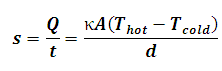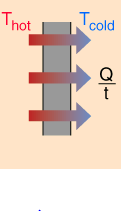# Rate of Heat Transfer Calculator

This Calctown Calculator calculates the rate of heat of transfer due to conduction.

K
K
W/mK
m2
m

#### Result

WClick here to view image

where

s = Q/t = rate of heat(Q) transfer

κ = thermal conductivity

T = temperature

d = path length of conduction

A = cross-sectional area